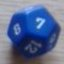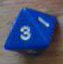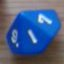Comparing two rolls for victory probability

## Comparing two rolls for victory probability

Return to topic list

 Wed Mar 14 22:04:08 2012   by   Hans I'm interested in comparing the results of two rolls and then calculating the probability that one beats the other.  For example, you roll 2d6 and I roll 2d6.  We both sum the totals of our rolls.  I win if my roll is greater than or equal to yours.  What is the probability I will win.  (I know this is simple to calculate without Troll, this is just an example).Intuitively, I think this should be...Sum 2d6 >= Sum 2d6But that doesn't work, because filters only work with singletons or constants on the left hand side.  This...d6 <= 2d6seems to work, albeit in a backwards kind of way.Can this be done?  If so, how?  And can it be done with more complicated rolls, for example, comparingsum largest 2 {4d8 drop 1, d10 drop 1}to sum largest 2 {3d8 drop 1, d6 drop 1}In case you are interested, this question is related to the new Marvel Heroic Roleplaying game Thu Mar 15 10:47:58 2012   by   Torben `>=` binds tighter than `sum`, so to get something like you want, you have to write `(sum 2d6) >= (sum 2d6)`.  If this is true, it returns the value of the second sum, otherwise it returns the empty collection of values.  You can read the probability of it being true as the accumulated probability of all non-empty values (55.633% in this case).You can do the same with the more complicated rolls, e.g.`(sum largest 2 {4d8 drop 1, d10 drop 1}) >= (sum largest 2 {3d8 drop 1, d6 drop 1})`If you only want to know win/lose, you can wrap it in an if-then-else:` if (sum largest 2 {4d8 drop 1, d10 drop 1}) >= (sum largest 2 {3d8 drop 1, d6 drop 1}) then "win" else "lose"` Tue Mar 27 22:17:34 2012   by   Hans That's great, thanks!

Return to topic list

New message:
 Topic: Posted by:

Type the values of the dice shown below:Return to topic list